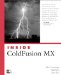# GetTickCount

"-->
` GetTickCount () `

#### Description

Determines the differences between the results of GetTickCount at successive points of page processing.

#### Example

` <cfset Begin = GetTickCount()>  <cfloop Index = i From = 1 To = 100>  <cfset counter = i>  </cfloop>  <cfset End = GetTickCount()>  <cfset totalTime = evaluate(End   Begin)>  <cfoutput>Total processing time was: #total Time# milliseconds</cfoutput>  `Inside Coldfusion MX
ISBN: 0735713049
EAN: 2147483647
Year: 2005
Pages: 579